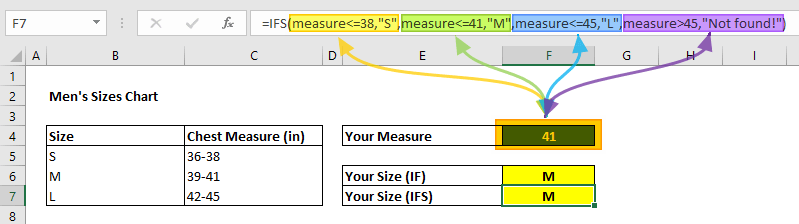The IFS function evaluates multiple conditions until finding first TRUE condition. It is intended to replace nested IF statements containing multiple IF functions. In this guide, we’re going to show you how to use the IFS function and also go over some tips and error handling methods.

# Supported versions

• Excel 2016 with Office 365 and above

# Syntax

IFS(logical_test1, value_if_true1, [logical_test2, value_if_true2], [logical_test3, value_if_true3],…)

# Arguments

 logical_test1 The first test for TRUE or FALSE evaluation. value_if_true1 The return value if logical_test1 result is TRUE. Can be left blank. logical_test2…logical_test127 Optional. The tests for evaluating for a TRUE or FALSE result. value_if_true2…value_if_true127 Optional. The return values if logical_testN returns TRUE. Can be left blank.

Example

We are going to be using named ranges in our examples to make the formulas easier to read, but this is not required.

formula evaluates every test-value pair until one of them returns a TRUE value. Since named range measure is 41 in our example, the 2nd test returns TRUE. Therefore, the function returns "M".# Tips

• You can test up to 127 different conditions.
• If you need a default value, enter TRUE for the last test. The function will evaluate the last TRUE test if there are no other successful tests.
• Use the SWITCH function if you have a single expression with multiple outcomes.

# Issues

## #N/A

If all tests returns FALSE, the function returns #N/A.

## #VALUE!

If a test returns a value other that TRUE or FALSE, the IFS function returns #VALUE!.